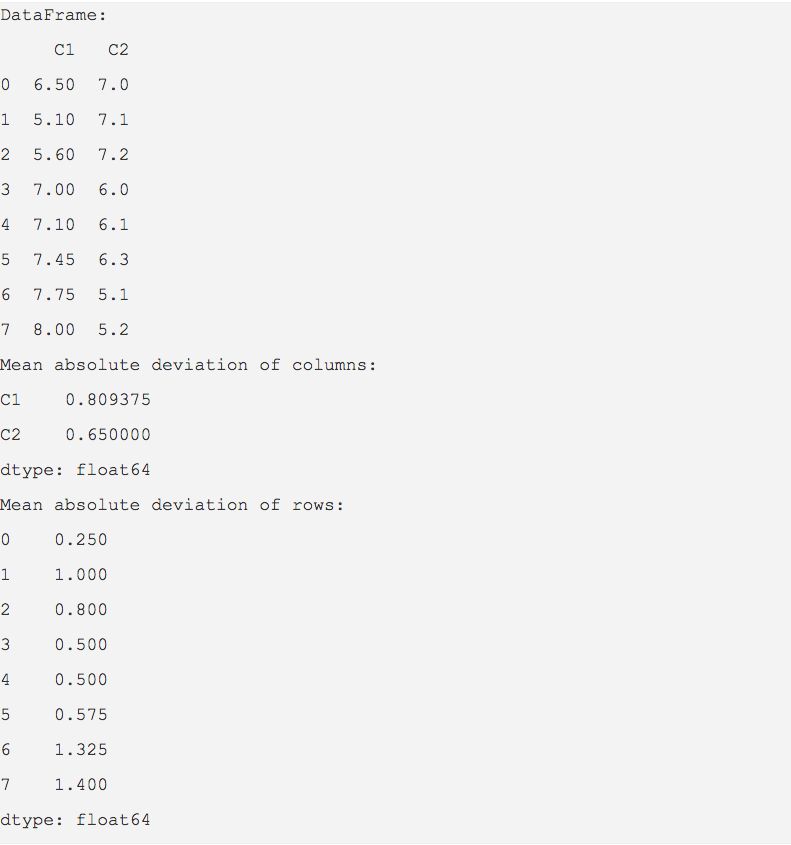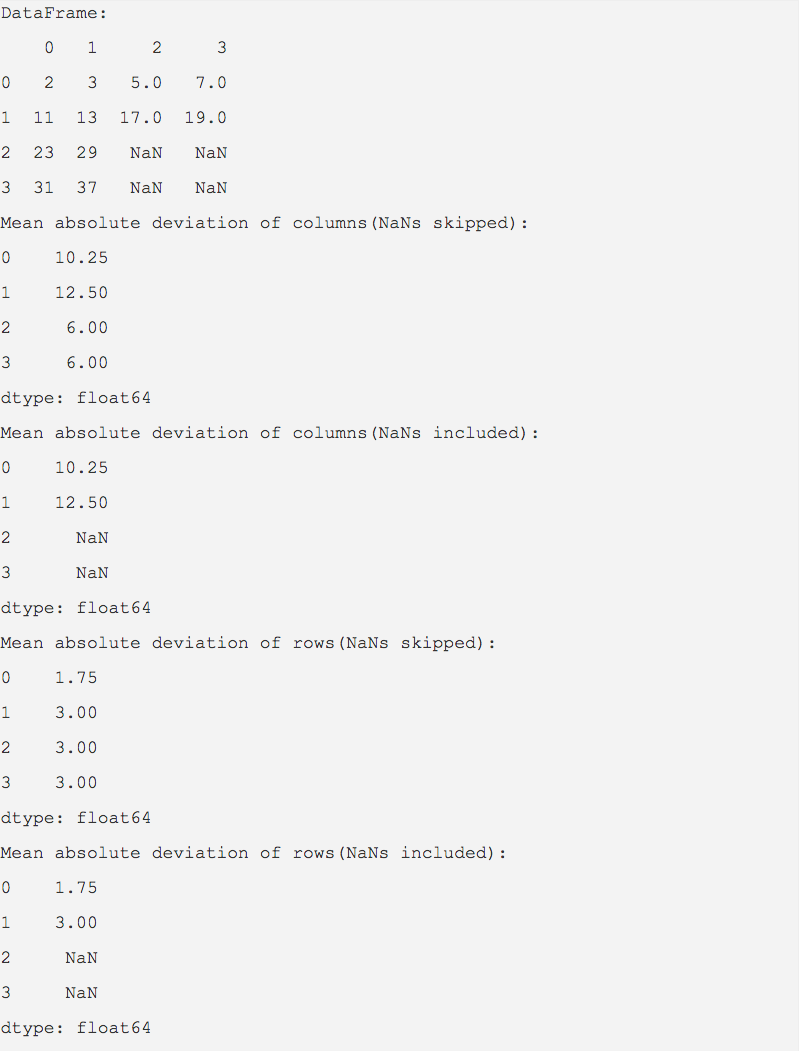# Find Mean Absolute Deviation Using Mad() Function In Pandas

## Overview:

• Mean Absolute Deviation (MAD) is computed as the mean of absolute deviation of data points from their mean.
• pandas DataFrame class has the method mad() that computes the Mean Absolute Deviation for rows or columns of a pandas DataFrame object.
• When mad() is invoked with axis = 0, the Mean Absolute Deviation is calculated for the columns. When axis=1, MAD is calculated for the rows.
• NaN values can be skipped using the optional Boolean parameter skipna. skipna=True skips the NaN values. skipna=False includes the NaN values. The default value for skipna is True.

## Example 1:

 import pandas as pd   dataSet = {"C1":(6.5, 5.1, 5.6, 7.0, 7.1, 7.45, 7.75, 8),            "C2":(7, 7.1, 7.2, 6, 6.1, 6.3, 5.1, 5.2)           } dataFrame = pd.DataFrame(data=dataSet); print("DataFrame:"); print(dataFrame)   # Calculate Mean Absolute Deviation of DataFrame columns mad = dataFrame.mad(); print("Mean absolute deviation of columns:"); print(mad);   # Calculate Mean Absolute Deviation of DataFrame rows mad = dataFrame.mad(axis=1); print("Mean absolute deviation of rows:"); print(mad);

## Output:## Example 2: